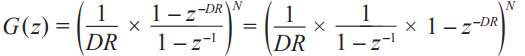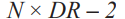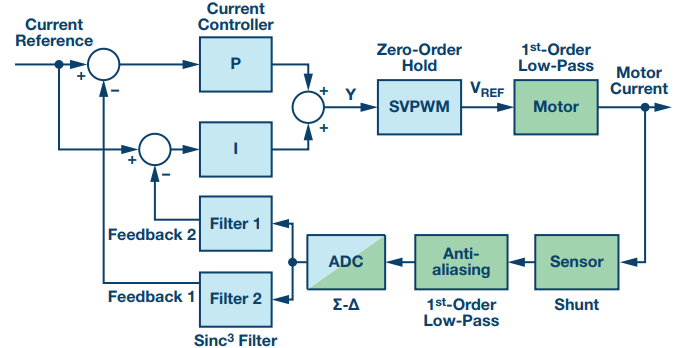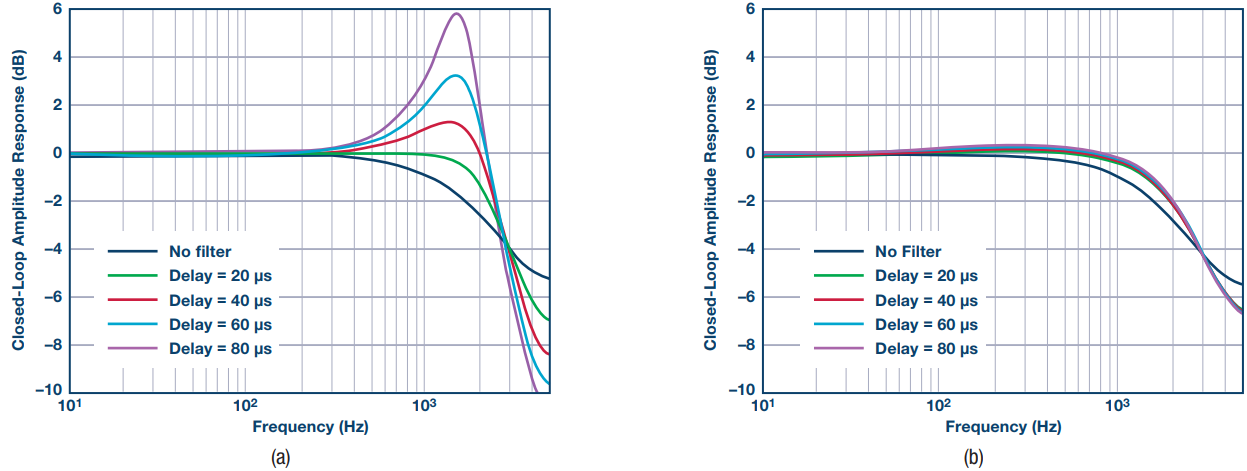# Sigma Delta Conversion Used for Motor Control

Ʃ-Δ analog-to-digital converters are widely used in motor drives where high signal integrity and galvanic isolation are required. While the Σ-Δ technology itself is well understood, the converters are often used in ways that fail to unlock the full potential of the technology. This article looks at Σ-Δ ADCs from an application point of view and discusses how to get the best performance in a motor drive.

### Introduction

When it comes to isolated phase current measurement in a 3-phase motor drive, there are several technologies to choose from. Three popular methods are outlined in Figure 1; an isolated sensor, such as a Hall effect or current transformer, combined with an amplifier, a resistive shunt combined with an isolation amplifier, and a resistive shunt combined with an isolating Σ-Δ ADC.

This article focuses on the highest performing method—Σ-Δ conversion. Typically, Σ-Δ ADCs target variable frequency motor drives and servo applications where high signal quality and galvanic isolation are required. Along with the ADC comes demodulation and filtering, which are typically handled by an FIR filter such as a 3rd-order sinc filter (sinc3).

A Σ-Δ ADC has the lowest possible resolution of 1 bit, but through oversampling, noise shaping, digital filtering, and decimation, very high signal quality can be achieved. The theory behind Σ-Δ ADCs and sinc filters is well understood and well documented,1, 2 so it will not be discussed in this article. Rather, the focus will be on how to get the best performance in a motor drive and how to utilize the performance in the control algorithms.

### Phase Current Measurement with Σ-Δ ADCs

When a 3-phase motor is fed by a switching voltage source inverter, the phase current can be seen as two components: an average component and a switching component, as seen in Figure 2. The top signal shows one phase current, the middle signal shows high-side PWM for the inverter phase-leg, and the lower signal shows the sample synchronizing signal from the PWM timer, PWM_SYNC. PWM_SYNC is asserted at the beginning and the center of a PWM cycle and so it aligns with the midpoint of the current and voltage ripple waveforms. For simplicity, it is assumed all three phases run with a duty cycle of 50%, which means there is only one rising slope and one falling slope of the current.Figure 2. Phase current assumes average value at the beginning and center of the PWM period.

For control purposes, only the average component of the current is of interest. The most common way to extract the average component is to sample the signal synchronized to PWM_SYNC. In these instances, the current assumes its average value so if there is tight control of the sampling instant, under sampling is possible without suffering from aliasing.

With conventional successive approximation (SAR) ADCs, sampling is done by a dedicated sample-and-hold circuit giving the user tight control of the sampling instant. Σ-Δ conversion, on the other hand, is a continuous sampling process and other means of extracting the average value of the current are needed. To get a better understanding of the problem at hand, a high level view of the Σ-Δ signal chain is helpful, as shown in Figure 3.

The first element is the converter itself. By sampling the analog signal at several MHz, the signal is converted into a 1-bit data stream. Furthermore, the converter shapes and pushes the quantization noise to higher frequencies. Following the converter is demodulation in the form of a filter and decimation. The filter converts the 1-bit signal into a multibit signal and the decimation process brings the update rate down so it matches that of the control algorithm. Filtering and decimation can be done in two stages, but a very common approach is to use a sinc filter which can do both in one stage. The sinc filter can be implemented in an FPGA, or as is becoming commonplace, the filter can also be a standard peripheral in a microprocessor.3 Regardless of how the sinc filter is implemented, 3rd-order (sinc3) is the most popular variant.

From a control point of view the ADC can be seen as ideal—a conversion rate of typically 10 MHz to 20 MHz introduces insignificant delay in a control loop with a bandwidth of a few kHz. The sinc3 filter, however, introduces a delay and makes it impossible to talk about one defined sampling instant. To get a better grasp on this, the filter’s representation in complex frequency domain, G(z), is helpful:DR is decimation rate and N is order. The filter is N integrators (1/(1 – z–1)) updated at sampling frequency and N differentiators (1 – zDR) updated at the decimation frequency (sampling frequency/DR). The filter has memory, which means the current output depends not only on current input but also on previous inputs and outputs. The behavior of the filter is best illustrated by plotting the filters impulse response:where y is the output sequence, x is the input sequence and h is the systems impulse response. Because a sinc filter is a linear and time invariant system, the impulse response h[n] can be used to determine the response to any input at any time. As an example, the impulse response of a 3rd-order sinc filter with a decimation rate of 5 is shown in Figure 4.Figure 4. Impulse response of 3rd-order sinc3 filter with decimation rate of 5.

As can be seen, the filter is a weighted sum, which gives more weight to samples at the center and less weight to samples at the beginning/end of the sequence. Due to the switching component of the phase currents this must be taken into account or feedback will suffer from aliasing. Fortunately, the impulse response is symmetrical, meaning the sinc filter gives equal weight to samples before and after the center pin. Furthermore, the switching component of the phase current is symmetrical around the point of average current. That is, if x equally spaced samples are taken before the instant of average current and added to x equally spaced samples taken after the instant of average current, the switching component sums to zero. This is achieved by aligning the center pin of the impulse response to the PWM_SYNC pulse as illustrated in Figure 5.

To align the impulse response to PWM correctly, the length of the impulse response must be known. The number of pins in impulse response of a 3rd-order filter is:Using this equation the length of impulse response in seconds can be calculated as:with tM being the period of the modulator clock. This time is important because it tells how long it takes a sample to propagate through the filter. The center pin of the impulse response is exactly halfway through the total filter length so the time it takes a sample to propagate halfway through must be:Therefore, if input sampling starts τd before PWM_SYNC and data from the filter is read τd after PWM_SYNC, alignment will be as shown in Figure 5. The start of sampling is controlled by the enable/disable of the modulator clock. Once enabled, the filter will remain synchronized with the PWM and there is no need to realign.

### Control Timing

By aligning the impulse response to PWM_SYNC it is possible to measure the phase current without aliasing but great care must be taken when data is read from the filter. The sinc filter is started τd before PWM_SYNC but it takes 2 × τd for data to propagate though the filter. In other words, it is vital to wait τd after PWM_SYNC before reading data from the filter. Only at this instant is the true average value of the current available. Compared to a SAR-based current measurement this makes a difference in control scheduling, as shown in Figure 6.

In the SAR case (a), the PWM_SYNC pulse triggers the ADC to do a number of samples and conversions. When data is ready for the control loop, an interrupt is generated and execution of the control loop can begin. In the Σ-Δ case, the wait is not for the ADC but to let data propagate through the sinc filter. When data is ready, an interrupt is generated, indicating that the control loop can be executed. To make an analogy, the conversion time of the SAR ADC is comparable to the duration of half an impulse response. How long half an impulse response is depends on modulation clock and decimation rate. A typical configuration of fM = 20 MHz and DR = 100, results in half an impulse response taking τd = 7.4 μs. While marginally longer than a fast SAR ADC, the numbers are comparable.

It should be noted that in a typical control system, the zero-order hold effect of the PWM timer far exceeds half an impulse response so the sinc filter does not greatly affect the loop timing.

### Σ-Δ ADCs Impact on Control Performance

With Σ-Δ ADCs, the user can freely choose between sinc filter delay and fidelity of the output data. At high decimation rates the delay is long but the signal quality is high. At low decimation rates the opposite is true. This flexibility is an advantage when it comes to design of motor control algorithms. Usually parts of the algorithm are sensitive to delay but less sensitive to the accuracy of the feedback. Other parts of the algorithm work with lower dynamics and benefit from accuracy but are less sensitive to delay. As an example, consider Figure 7 (a) showing a conventional proportional-integral controller (PI).4, 5 Both the P part and I part operate with the same feedback signal, meaning the signal must have dynamics that fit both control paths. However, it is possible to split the P path and I path, as shown in Figure 7 (b) and from this stage it is a small step to Figure 7 (c) where the P path and I path are separate and operate with feedback signals that have different dynamic properties.Figure 7. PI controller implementations. (a) Conventional implementation, (b) split P path and I path, (c) split P path and I path and split feedback.

The task of the P part is to suppress fast load changes and fast speed changes, but precision is not a main concern. In other words, the P part would benefit from a sinc filter with a low decimation rate and short delay. The task of the I part is ensure stable and accurate steady state performance, which requires high precision. Therefore, the I part would benefit from a sinc filter with a high decimation rate and longer delay. This leads to an implementation as shown in Figure 8.Figure 8. Dual sinc filter and seperate P path and I path of current controller.

The motor phase current is measured by a sensor (shunt) and through an antialiasing filter fed to the Σ-Δ ADC. The 1-bit data stream is then input into two sinc filters—one that is tuned for the P controller and one that tuned for the I controller. In Figure 8, the Clark and Park transformations are omitted for simplicity. However, current control is done in a rotating dq frame.

To assess the impact of splitting the current feedback into two paths, a stability analysis of the closed-loop has been performed. For the traditional Z-domain analysis, the sinc filter poses a problem. It introduces a delay, which for any practical decimation rate is less than one sample period. For example, if a system running at fsw = 10 kHz, the delay of the filter will be less than 100 μs. From the control loop’s point of view, the sinc block is a fractional delay filter. To model a fractional delay, the sinc filter was approximated by an all-pass filter. At lower frequencies—up to about half the Nyquist frequency—the approximation is accurate but at a higher frequency there is some deviation from the ideal filter. However, the purpose here is to get an understanding of how dual feedback affects loop stability and for this the approximation serves well.

For comparison, Figure 9 (a) shows the closed-loop amplitude response when only one sinc filter is used in the feedback path (no dual feedback). The switching frequency fsw is 10 kHz, setting the Nyquist frequency at 5 kHz. With these system parameters, the closed-loop response is plotted for sinc filter group delays ranging from 0 μs to 80 μs. Note, group delay relates directly to decimation rate. As expected, low decimation rate and group delay has little effect on closed-loop stability, but as the delay is increased, the system becomes less and less damped.Figure 9. Effect of dual feedback on current control performance, (a) with shared sinc filters for P controller and I controller, (b) with separate sinc filters for P controller and I controller.

Now when splitting the feedback into separate paths for the P controller and I controller, Figure 9 (b) is obtained. In this case the decimation rate of the sinc filter for the P controller is fixed to give a group delay of 10 μs. Only the decimation rate of the I controller is varied.

As seen in Figure 9 (b), increasing the delay for the I controller has very little effect on closed-loop stability. As discussed, these properties can be utilized to increase the dynamic, as well as steady state performance of the loop.

In this article the algorithm using split feedback is a PI controller. However, this is only one example and most control systems have several algorithms that would benefit from having their feedback tuned, according to the dynamic and precision requirements. A few examples are flux observers, feed forward controllers, and differential parts of a PID controller.

### Filtering Technique

A filter has a finite attenuation and some switching noise from turning on/off the IGBTs of the inverter will pass through filter. This section will explore a technique that helps eliminate switching noise from the current feedback.

If a motor is driven by a voltage source inverter using standard space vector modulation (SVPWM6) the noise spectrum of the phase current will be characterized by side bands centered around integer multiples of the switching frequency. For example, if 10 kHz switching frequency is used, there would be high levels of noise around n × 10 kHz with n being an integer. A typical spectrum is shown by the green curve in Figure 10. These sidebands introduce noise in the current feedback and effective attenuation is therefore desirable.Figure 10. Power spectrum of phase current (green) along with sinc filter amplitude response (purple).

The location of the poles/zeros of the sinc filter are determined by the decimation rate and modulation frequency. That means the user has freedom to tune the frequency response of the filter to best fit the application. An amplitude response of a 3rd-order sinc filter is shown by the purple curve in Figure 10. As expected, the amplitude trails off at higher frequencies but the amplitude also has characteristic notches where the attenuation approaches infinity. The notches are at frequencies determined by modulator clock and decimation rate:If notches are put at the same frequencies as the sidebands of the phase current spectrum, the inverter switching noise is very effectively attenuated. As an example, consider a case where the inverter switching frequency fsw is 10 kHz, the ADC modulator clock fM is 8 MHz and decimation rate DR is 800. This puts the notches at n × 10 kHz and the response is as shown in Figure 10. Note how each sideband is attenuated by a notch.

Some HW implementations of the sinc filter will not support decimation rates high enough to place a pole/zero around the PWM frequency. Furthermore, the filter group delay associated with such high decimation rates may be unacceptable. In the example used in Figure 10, the decimation rate of 800 and modulation frequency of 8 MHz gives a delay of 150 μs.

Another approach is to let the sinc filter run with a lower decimation rate and then do postprocessing of the data in software. Still assuming fsw = 10 kHz and fM is 8 MHz, a possible approach is to let the HW sinc filter run with a decimation rate of 200, which results in a data rate of 8 MHz/200 is 40 kHz. Now this data rate is too high for the motor control algorithm, which makes it possible to introduce a SW filter that takes the data rate down to 10 kHz. An example of such a filter is a 1st-order sinc filter with a decimation rate of 4 (effectively a moving average of over four samples). This configuration is shown in Figure 11.

Since the HW filter outputs data at a rate higher than needed by the control algorithm, the SW filter adds little delay to the signal—much less than what would be the case if the HW filter is used directly to decimate down to the updated rate of the control algorithm. Furthermore, the sinc1 filter still puts a notch at all the sidebands of the phase current spectrum. Therefore, the benefits of highly attenuating the switching noise from the inverter still exists.

The filtering technique can be combined with the split feedback path. Since the combination of an HW and SW sinc filter gives very high attenuation but adds some phase to the current feedback, the technique lends itself best to the I path.

### Implementation and Test

The concepts described in this article have been implemented and verified on a 400 V motor control platform from Analog Devices, see Figure 12. The power board features universal 110 VAC/230 VAC input voltage, boost power factor correction, 3-phase IGBT inverter rated at 5 ARMS continuous. The motor is a Kollmorgen AKM22 3-phase PM servo motor with incremental encoder feedback. The Σ-Δ ADC used for current feedback is AD7403. The Σ-Δ ADCs interface directly to a processor, ADSP-CM408, which has built-in sinc filters that support the measuring techniques described in this article. For further information refer to.7

### Conclusion

Despite the lack of defined sampling instant, Σ-Δ conversion can be used to measure motor currents without aliasing effects. The technique described gives proper alignment of sinc filter impulse response to the PWM signals.

Using a PI controller as an example, it was shown that parallel sinc filters can be tuned to match the requirement of the control algorithm. The result is improved bandwidth and steady state performance.

Finally, it was discussed how carefully positioning the sinc filter zeros can help eliminate switching noise in the current feedback. All the concepts were implemented and verified on a 400 V inverter driving a permanent magnet motor.

### References

1 Application Note AN-283. Sigma-Delta ADCs and DACs, Analog Devices.

2 Hogenauer, Eugene B. “An Economical Class of Digital Filters for Decimation and Interpolation.” IEEE Transactions on Acoustics, Speech, and Signal Processing. April, 1981.

4 Klarenbach, Christoph, Jens Onno Krah, and Heiko Schmirgel. “Design of Fast and Robust Current Controllers for Servo Drives Based on Space Vector Modulation.” PCIM Europe. 2011.

5 Krah, Jens Onno. “Closed-Loop Control Method and Closed-Loop Control Device with Multi-Channel Feedback.” US 20100141198 A1.

6 Holtz, Joachim. “Pulsewidth Modulation—A Survey.” IEEE Transactions on Industrial Electronics, Vol. 39.